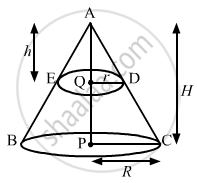Advertisement Remove all ads

# Choose the Correct Answer of the Following Question: a Solid Right Circular Cone is Cut into Two Parts at the Middle of Its Height by a Plane Parallel to Its - Mathematics

MCQ

Choose the correct answer of the following question:

A solid right circular cone is cut into two parts at the middle of its height by a plane parallel to its base. The ratio of the volume of the smaller cone to the whole cone is

#### Options

• 1 : 2

• 1 : 4

• 1 : 6

• 1 : 8

Advertisement Remove all ads

#### SolutionLet the radii of the smaller and given cones be r and R, respectively; and their heights b and H respectively

We have,

H = 2h   .........(i)

In ΔAQD and ΔAPC,

∠QAD = ∠PAC      (common angle)

∠AQD = ∠APC = 90°

So, by AA citeria

Δ AQD ˜ Δ APC

=> "AQ"/"AP" = "QD"/"PC"

=> "h"/"H" = "r"/"R"

=> "h"/"2h" = "r"/"R"

=>1/2 = "r"/"R"

⇒ R = 2r        ........(ii)

Now,

The ratio of the smaller cone to the whole cone="Volume of the smaller cone"/"Volume of the whole cone"

= ((1/3pi"r"^2"h"))/((1/3pi"R"^2H))

=("r"/"R")^2 xx ("h"/"H")

=("r"/"2r")^2xx("h"/"2h")          [Using (i) and (ii)]

=(1/2)^2 xx (1/2)

=1/8

= 1 : 8

Hence, the correct answer is option (d).

Is there an error in this question or solution?
Advertisement Remove all ads

#### APPEARS IN

RS Aggarwal Secondary School Class 10 Maths
Chapter 19 Volume and Surface Area of Solids
Multiple Choice Questions | Q 21 | Page 920
Advertisement Remove all ads
Advertisement Remove all ads
Share
Notifications

View all notifications

Forgot password?